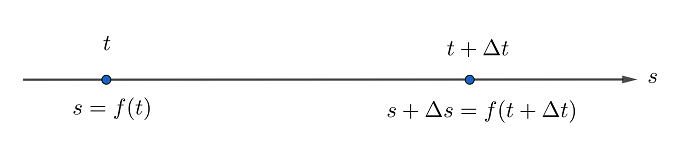安卓手机扫描二维码安装App﻿$v=\dfrac{\Delta s}{\Delta t}$﻿ ﻿$=\dfrac{f(t+\Delta t) -f(t)}{\Delta t}$﻿

﻿$t$﻿ 时刻的速度（瞬时速度）是位置关于时间的导数

﻿$v_{(t)}=\dfrac{ds}{dt}$﻿﻿$=\lim\limits_{ \Delta \to 0} \dfrac{f(t+\Delta t) -f(t)}{\Delta t}$﻿

﻿$t$﻿ 时刻的速率是速度的绝对值

﻿$a_{(t)}=\dfrac{dv}{dt}=\dfrac{d^2s}{dt^2}$﻿

﻿$j_(t)=\dfrac{da}{dt}=\dfrac{d^3s}{dt^3}$﻿

﻿$s=\dfrac{1}{2} g t^2$﻿ ，其中 ﻿$g$﻿ 是重力加速度，那么自由落体的急推是多少？苹果手机扫描二维码安装App